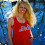## Tuesday, October 27, 2015

### 27 44 93 118 | The Death of Father of Starting Pitcher for Game 1 of 111th World Series, Edinson Volquez

Edinson = 5+4+9+5+1+6+5 = 35/44
Volquez = 4+6+3+8+3+5+8 = 37
Edinson Volquez = 72/81

Edinson = 5+4+9+14+19+15+14 = 80
Volquez = 22+15+12+17+21+5+26 = 118
Edinson Volquez = 198

Notice the Gematria of '44' and '118' within his name.  These are the numbers for 'kill' and 'death'.

Daniel = 4+1+5+9+5+3 = 27
Volquez = 4+6+3+8+3+5+8 = 37
Daniel Volquez = 64

Daniel = 4+1+14+9+5+12 = 45
Volquez = 22+15+12+17+21+5+26 = 118
Daniel Volquez = 163 (Dead at 63)

DV = 422 or 44

Again, the numbers 44 and 118 have much to do with killing and death.  It seems strange this man would drop dead with heart disease moments before his son pitched in the opening game of the World Series.

Chris = 3+8+9+9+1= 30/39
Young = 7+6+3+5+7 = 28
Chris Young = 58/67 (Freemasonry = 58/67)

Chris = 3+8+18+9+19 = 57
Young = 25+15+21+14+7 = 82
Chris Young = 139 (Freemasonry = 139)

His life numbers are fitting for facing New York, the land of 39, 93 and 111.  Recall, the Royals have 39 and 93 connections of their own.

7/3/1983 = 7+3+19+83 = 112
7/3/1983 = 7+3+(1+9+8+3) = 31
7/3/1983 = 7+3+1+9+8+3 = 31
7/3/83 = 7+3+83 = 93

This ritualistic killing must stop.

#### 1 comment:

1.The media can never keep its stories straight. First, Volquez was informed about his father after he was done pitching. Then he was informed before the game even started....